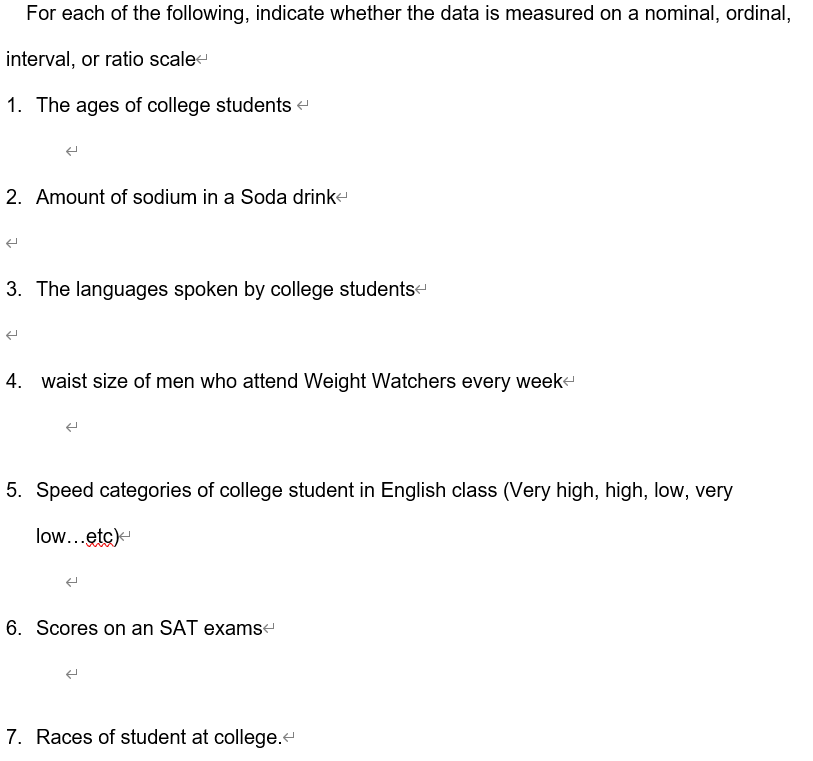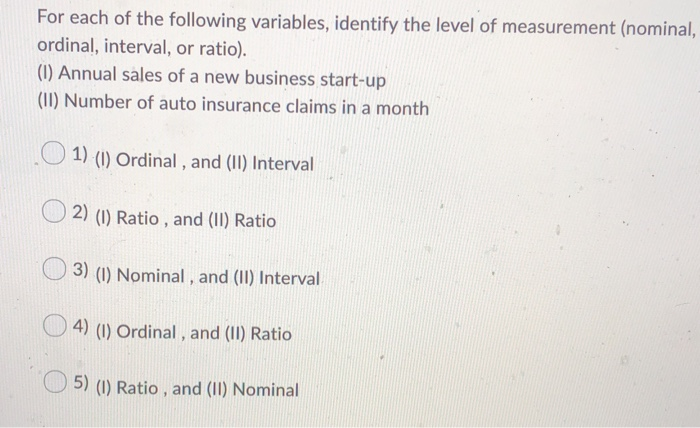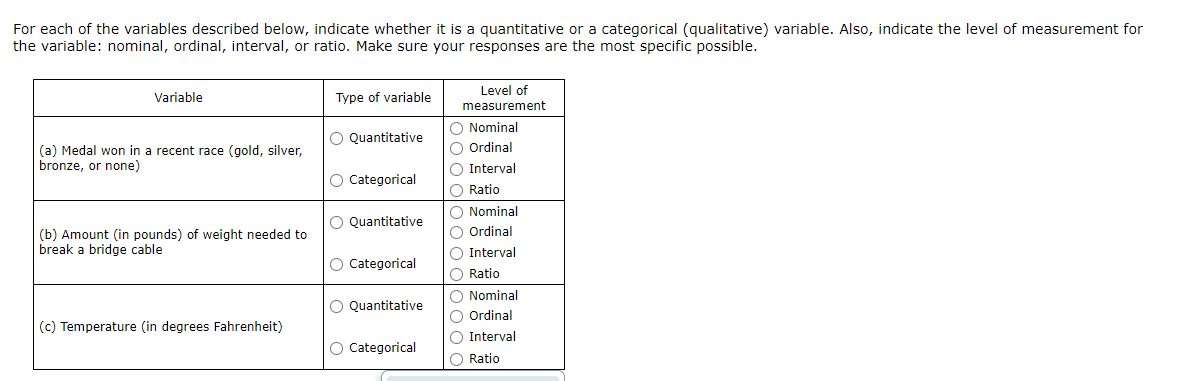Question

# Indicate whether each measure is a nominal, ordinal, or interval/ratio measure. Explain the reasons for your...

Indicate whether each measure is a nominal, ordinal, or interval/ratio measure. Explain the reasons for your choices: [3 points each, 15 points]

a. inches in a yardstick:

b. Virginia driver’s license customer ID:

c.dollars as a measure of income:

d.order of finish in a horse race:

e.intelligence test scores:

Nominal scale : Nominal scale consist of two or more named categories into which the object are classified .

Ordinal Scale : Ordinal scale of measurement gives number to groups of objects using suitable characteristics so that order arrangement of group is possible

Ratio scale : Ratio scale of measurement has equal units of measurement and those are taken from true zero .

a. inches in a yardstick: This is example of ratio scale because inches are measure from true value 0 .

b. Virginia driver’s license customer ID: This is example of nominal scale because the number assign or label to the license customer id as per the approach and the numbers are interchangeable .

c. dollars as a measure of income: This is example of ordinal scale because the group of income measure as dollar is such as poor , middle class , rich .

d. order of finish in a horse race: This is example of Ordinal scale because the number assign or label in order of finish in a horse race .

e. .intelligence test scores: A)intelligence test score is purely a number . This is example of ratio scale because it is measure from true value 0. and if a student got 30 marks and another student got 60 marks . then second student get exactly double marks then that of first student . B) In some type of examination there is a scheme of negative marks also , In that case , Interval scale is used .

#### Earn Coins

Coins can be redeemed for fabulous gifts.

Similar Homework Help Questions
• ### For each type of data below, indicate whether it is nominal, ordinal, interval, or ratio. Please...

For each type of data below, indicate whether it is nominal, ordinal, interval, or ratio. Please also indicate whether it is discrete or continuous. (1) Number of chairs sold in a week. (2) Name of your college dorm. (3) Elevation (feet above sea level) (4) Year in college (Junior, Senior, etc.) (5) Time on a 12:00 hour clock with hands.

• ### For each of the following, indicate whether the data is measured on a nominal, ordinal, interval,...For each of the following, indicate whether the data is measured on a nominal, ordinal, interval, or ratio scale- 1. The ages of college students 2. Amount of sodium in a Soda drink 3. The languages spoken by college students 4. waist size of men who attend Weight Watchers every week 5. Speed categories of college student in English class (Very high, high, low, very low...etc) 6. Scores on an SAT exams- 7. Races of student at college.“

• ### (1) For each of the following, indicate whether the data is measured on a nominal, ordinal,...

(1) For each of the following, indicate whether the data is measured on a nominal, ordinal, interval, or ratio scale. _______ a) heights of college students _______ b) amount of sugar in a cheese _______ c) borough of origin of students _______ d) amount of snow that falls in NY in January _______ e) languages spoken by college students _______ f) waist size of men who attend Weight Watchers every week _______ g) wrist sizes in inches of NFL players...

• ### For each item, indicate whether the survey question’s level of measurement is: Nominal (N), Ordinal (O),...

For each item, indicate whether the survey question’s level of measurement is: Nominal (N), Ordinal (O), Interval (I) or Ratio (R). 1) Please rank each brand in terms of your preference. Place a "1" by your first choice, a "2" by your second choice, etc... _______Arid ________Right Guard   ________ Mennen ( ) Nominal ( ) Ordinal ( ) Interval ( ) Ratio 2) How many hours do you spend online per week? _______ ( ) Nominal ( ) Ordinal (...

• ### Determine whether the following possible responses should be classified as ratio, interval, nominal or ordinal data...Determine whether the following possible responses should be classified as ratio, interval, nominal or ordinal data ﹀ | 1, Amount of time you spend per week on your homework 2. Lily's travel time from her dorm to the student union at the University of Virginia 3. Number of cars in a parking lot 4. Whether you are a US citizen

• ### Determine whether the following possible responses should be classified as ratio, interval, nominal or ordinal data...Determine whether the following possible responses should be classified as ratio, interval, nominal or ordinal data ﹀ | 1, Amount of time you spend per week on your homework 2. Lily's travel time from her dorm to the student union at the University of Virginia 3. Number of cars in a parking lot 4. Whether you are a US citizen

• ### For each of the following variables, indentify the level of measurement (nominal, ordinal, interval, or ratio)....

For each of the following variables, indentify the level of measurement (nominal, ordinal, interval, or ratio). (I) Product defect rates for a new a cell phone (II) Film genre (e.g. comedy, horror, drama, etc) Answers 1) (I) Ratio, and (II)Nominal 2) (I) Interval, and (II) Nominal 3) (I) Ratio, and (II) Interval 4) (I) Interval, and (II) Ordinal 5) (I) ordinal, and (II) Ordinal

• ### For each of the following variables, identify the level of measurement (nominal, ordinal, interval, or ratio)....For each of the following variables, identify the level of measurement (nominal, ordinal, interval, or ratio). (1) Annual sales of a new business start-up (II) Number of auto insurance claims in a month 1) (1) Ordinal , and (II) Interval 2) (1) Ratio , and (II) Ratio 3) (1) Nominal, and (11) Interval O4) (1) Ordinal, and (II) Ratio 5) (0) Ratio , and (II) Nominal

• ### For each of the variables described below, indicate whether it is a quantitative or a categorical...For each of the variables described below, indicate whether it is a quantitative or a categorical (qualitative) variable. Also, indicate the level of measurement for the variable: nominal, ordinal, interval, or ratio. Make sure your responses are the most specific possible. Variable Type of variable O Quantitative (a) Medal won in a recent race (gold, silver, bronze, or none) O Categorical O Quantitative Level of measurement O Nominal Ordinal Interval O Ratio O Nominal Ordinal Interval Ratio Nominal O Ordinal...

• ### For each of the variables described below, indicate whether it is a quantitative or a categorical...

For each of the variables described below, indicate whether it is a quantitative or a categorical (qualitative) variable. Also, indicate the level of measurement for the variable: nominal, ordinal, interval, or ratio. Make sure your responses are the most specific possible. Variable Type of variable Level of measurement (a) Dosage (in milligrams) of medication Quantitative Nominal Ordinal Categorical Interval Ratio (b) Customer satisfaction rating (very satisfied, somewhat satisfied, somewhat dissatisfied, or very dissatisfied) Quantitative Nominal Ordinal Categorical Interval Ratio (c)...